# Red-Black Trees | Top-Down Insertion

In Bottom-Up insertion of Red-Black Trees, “simple” Binary Search Tree insertion is used, followed by correction of the RB-Tree Violations on the way back up to the root. This can be done easily with the help of recursion. While in Top-Down Insertion, the corrections are done while traversing down the tree to the insertion point. When the actual insertion is done, no further corrections are needed, so no need to traverse back up the tree.

Therefore, the goal of Top-Down insertion is to traverse from the root to the insertion point in such a way that RB properties are maintained. This iterative approach thus makes Top-Down insertion faster than Bottom-Up insertion.

The two basic operations to perform for fixing violations and balancing are-

• Recoloring
• Rotation
• Following is the detailed Algorithm
The main goal of this algorithm is to create an insertion point at which the parent of the new node is Black, or the uncle of the new node is black.

Let N be the new node to be inserted.

1. If Y and Z are Black:
2.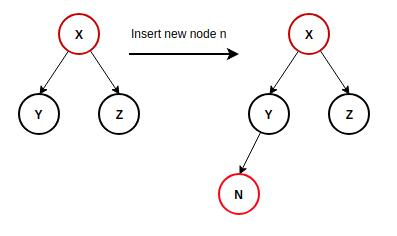perform Simple BST insertion. Insert new node N as the left/right child of Y OR Z and make the colour of the newly inserted node as red.

3. If X’s Parent is Black:
4.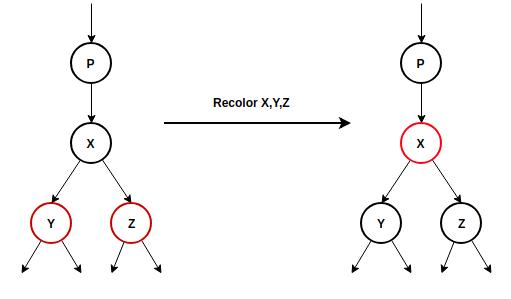Then Recolor X, Y, Z and continue Down the tree.

5. X’s Parent P is Red, Grandparent is Black and X and P are both left OR right children of Grandparent G:
6.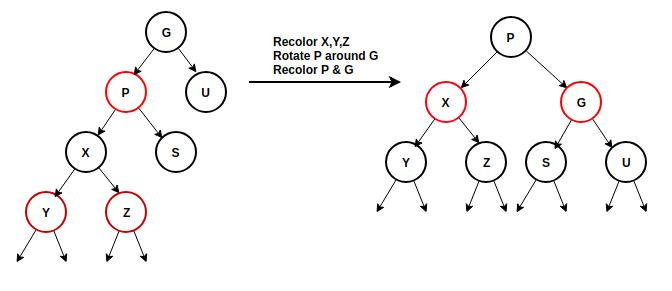• Recolor X, Y, Z
• Rotate P around G
• Color P black
• Color G red

If violations exist continue with further cases.

7. X’s Parent is Red, Grandparent is Black and X and P are opposite children of Grandparent G
8.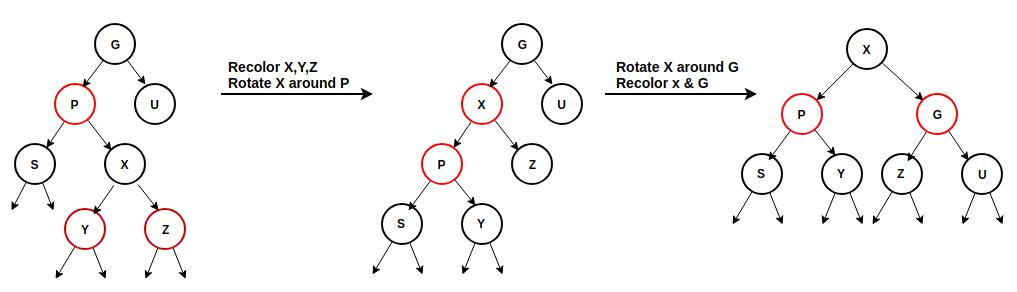• Recolor X, Y, Z
• Rotate X around P
• Rotate X around G
• Recolor X and G

Insert New Node N at the required Position.

Example :
Insert Node 3 in the RB-Tree below –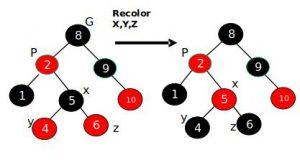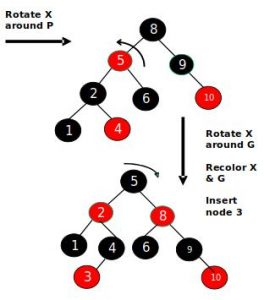Below the implementation of the following approach:

## Java

 `// Java implementation for Top-Down ` `// Red-Black Tree Insertion creating ` `// a red black tree and storing an ` `// English sentence into it using Top ` `// down insertion approach ` ` `  `import` `static` `java.lang.Integer.max; ` ` `  `// Class for performing ` `// RBTree operations ` `public` `class` `RbTree { ` `    ``TreeNode Root = ``null``; ` ` `  `    ``// Function to calculate ` `    ``// the height of the tree ` `    ``int` `HeightT(TreeNode Root) ` `    ``{ ` `        ``int` `lefth, righth; ` ` `  `        ``if` `(Root == ``null` `            ``|| (Root.children == ``null` `                ``&& Root.children[``1``] == ``null``)) { ` `            ``return` `0``; ` `        ``} ` `        ``lefth = HeightT(Root.children[``0``]); ` `        ``righth = HeightT(Root.children[``1``]); ` ` `  `        ``return` `(max(lefth, righth) + ``1``); ` `    ``} ` ` `  `    ``// Function to check if ` `    ``// dir is equal to 0 ` `    ``int` `check(``int` `dir) ` `    ``{ ` `        ``return` `dir == ``0` `? ``1` `: ``0``; ` `    ``} ` ` `  `    ``// Function to check if a ` `    ``// node's color is red or not ` `    ``boolean` `isRed(TreeNode Node) ` `    ``{ ` `        ``return` `Node != ``null` `            ``&& Node.color.equals(``"R"``); ` `    ``} ` ` `  `    ``// Function to perform ` `    ``// single rotation ` `    ``TreeNode SingleRotate(TreeNode Node, ` `                          ``int` `dir) ` `    ``{ ` `        ``TreeNode temp ` `            ``= Node.children[check(dir)]; ` `        ``Node.children[check(dir)] ` `            ``= temp.children[dir]; ` `        ``temp.children[dir] = Node; ` `        ``Root.color = ``"R"``; ` `        ``temp.color = ``"B"``; ` ` `  `        ``return` `temp; ` `    ``} ` ` `  `    ``// Function to perform double rotation ` `    ``TreeNode DoubleRotate(TreeNode Node, ` `                          ``int` `dir) ` `    ``{ ` `        ``Node.children[check(dir)] ` `            ``= SingleRotate(Node.children[check(dir)], ` `                           ``check(dir)); ` `        ``return` `SingleRotate(Node, dir); ` `    ``} ` ` `  `    ``// Function to insert a new ` `    ``// node with given data ` `    ``TreeNode Insert(RbTree tree, ` `                    ``String data) ` `    ``{ ` `        ``if` `(tree.Root == ``null``) { ` `            ``tree.Root ` `                ``= ``new` `TreeNode(data); ` `            ``if` `(tree.Root == ``null``) ` `                ``return` `null``; ` `        ``} ` `        ``else` `{ ` ` `  `            ``// A temporary root ` `            ``TreeNode temp = ``new` `TreeNode(``""``); ` ` `  `            ``// Grandparent and Parent ` `            ``TreeNode g, t; ` `            ``TreeNode p, q; ` ` `  `            ``int` `dir = ``0``, last = ``0``; ` ` `  `            ``t = temp; ` ` `  `            ``g = p = ``null``; ` ` `  `            ``t.children[``1``] = tree.Root; ` ` `  `            ``q = t.children[``1``]; ` `            ``while` `(``true``) { ` ` `  `                ``if` `(q == ``null``) { ` ` `  `                    ``// Inserting root node ` `                    ``q = ``new` `TreeNode(data); ` `                    ``p.children[dir] = q; ` `                ``} ` ` `  `                ``// Sibling is red ` `                ``else` `if` `(isRed(q.children[``0``]) ` `                         ``&& isRed(q.children[``1``])) { ` ` `  `                    ``// Recoloring if both ` `                    ``// children are red ` `                    ``q.color = ``"R"``; ` `                    ``q.children[``0``].color = ``"B"``; ` `                    ``q.children[``1``].color = ``"B"``; ` `                ``} ` ` `  `                ``if` `(isRed(q) && isRed(p)) { ` ` `  `                    ``// Resolving red-red ` `                    ``// violation ` `                    ``int` `dir2; ` `                    ``if` `(t.children[``1``] == g) { ` `                        ``dir2 = ``1``; ` `                    ``} ` `                    ``else` `{ ` `                        ``dir2 = ``0``; ` `                    ``} ` ` `  `                    ``// If children and parent ` `                    ``// are left-left or ` `                    ``// right-right of grand-parent ` `                    ``if` `(q == p.children[last]) { ` `                        ``t.children[dir2] ` `                            ``= SingleRotate(g, ` `                                           ``last == ``0` `                                               ``? ``1` `                                               ``: ``0``); ` `                    ``} ` ` `  `                    ``// If they are opposite ` `                    ``// childs i.e left-right ` `                    ``// or right-left ` `                    ``else` `{ ` `                        ``t.children[dir2] ` `                            ``= DoubleRotate(g, ` `                                           ``last == ``0` `                                               ``? ``1` `                                               ``: ``0``); ` `                    ``} ` `                ``} ` ` `  `                ``// Checking for correct ` `                ``// position of node ` `                ``if` `(q.data.equals(data)) { ` `                    ``break``; ` `                ``} ` `                ``last = dir; ` ` `  `                ``// Finding the path to ` `                ``// traverse [Either left ` `                ``// or right ] ` `                ``dir = q.data.compareTo(data) < ``0` `                          ``? ``1` `                          ``: ``0``; ` ` `  `                ``if` `(g != ``null``) { ` `                    ``t = g; ` `                ``} ` ` `  `                ``// Rearranging pointers ` `                ``g = p; ` `                ``p = q; ` `                ``q = q.children[dir]; ` `            ``} ` ` `  `            ``tree.Root = temp.children[``1``]; ` `        ``} ` ` `  `        ``// Assign black color ` `        ``// to the root node ` `        ``tree.Root.color = ``"B"``; ` ` `  `        ``return` `tree.Root; ` `    ``} ` ` `  `    ``// Print nodes at each ` `    ``// level in level order ` `    ``// traversal ` `    ``void` `PrintLevel(TreeNode root, ``int` `i) ` `    ``{ ` `        ``if` `(root == ``null``) { ` `            ``return``; ` `        ``} ` ` `  `        ``if` `(i == ``1``) { ` `            ``System.out.print(``"| "` `                             ``+ root.data ` `                             ``+ ``" | "` `                             ``+ root.color ` `                             ``+ ``" |"``); ` ` `  `            ``if` `(root.children[``0``] != ``null``) { ` `                ``System.out.print(``" "` `                                 ``+ root.children[``0``].data ` `                                 ``+ ``" |"``); ` `            ``} ` `            ``else` `{ ` `                ``System.out.print(``" "` `                                 ``+ ``"NULL"` `                                 ``+ ``" |"``); ` `            ``} ` `            ``if` `(root.children[``1``] != ``null``) { ` `                ``System.out.print(``" "` `                                 ``+ root.children[``1``].data ` `                                 ``+ ``" |"``); ` `            ``} ` `            ``else` `{ ` `                ``System.out.print(``" "` `                                 ``+ ``"NULL"` `                                 ``+ ``" |"``); ` `            ``} ` ` `  `            ``System.out.print(``" "``); ` ` `  `            ``return``; ` `        ``} ` ` `  `        ``PrintLevel(root.children[``0``], ` `                   ``i - ``1``); ` `        ``PrintLevel(root.children[``1``], ` `                   ``i - ``1``); ` `    ``} ` ` `  `    ``// Utility Function to ` `    ``// perform level order ` `    ``// traversal ` `    ``void` `LevelOrder(TreeNode root) ` `    ``{ ` `        ``int` `i; ` ` `  `        ``for` `(i = ``1``; ` `             ``i < HeightT(root) + ``1``; ` `             ``i++) { ` `            ``PrintLevel(root, i); ` `            ``System.out.print(``"\n\n"``); ` `        ``} ` `    ``} ` `} ` ` `  `// Class for representing ` `// a node of the tree ` `class` `TreeNode { ` ` `  `    ``// Class variables ` `    ``String data, color; ` `    ``TreeNode children[]; ` ` `  `    ``public` `TreeNode(String data) ` `    ``{ ` `        ``// Color R- Red ` `        ``// and B - Black ` `        ``this``.data = data; ` `        ``this``.color = ``"R"``; ` `        ``children ` `            ``= ``new` `TreeNode[``2``]; ` `        ``children[``0``] = ``null``; ` `        ``children[``1``] = ``null``; ` `    ``} ` `} ` ` `  `// Driver Code ` `class` `Driver { ` `    ``public` `static` `void` `main(String[] args) ` `    ``{ ` `        ``// Tree Node Representation ` `        ``// ------------------------------------------- ` `        ``// DATA | COLOR | LEFT CHILD | RIGHT CHILD | ` `        ``// ------------------------------------------- ` ` `  `        ``RbTree Tree = ``new` `RbTree(); ` `        ``String Sentence, Word; ` `        ``Sentence = ``"old is gold"``; ` `        ``String Word_Array[] ` `            ``= Sentence.split(``" "``); ` ` `  `        ``for` `(``int` `i = ``0``; ` `             ``i < Word_Array.length; ` `             ``i++) { ` `            ``Tree.Root ` `                ``= Tree.Insert(Tree, ` `                              ``Word_Array[i]); ` `        ``} ` ` `  `        ``// Print Level Order Traversal ` `        ``System.out.println(``"The Level"` `                           ``+ ``"Order Traversal"` `                           ``+ ``"of the tree is:"``); ` `        ``Tree.LevelOrder(Tree.Root); ` `        ``System.out.println(``"\nInserting a"` `                           ``+ ``" word in the tree:"``); ` `        ``Word = ``"forever"``; ` `        ``Tree.Root = Tree.Insert(Tree, ` `                                ``Word); ` ` `  `        ``System.out.println(``""``); ` `        ``Tree.LevelOrder(Tree.Root); ` `    ``} ` `} `

## C#

 `// C# implementation for Top-Down ` `// Red-Black Tree Insertion creating ` `// a red black tree and storing an ` `// English sentence into it using Top ` `// down insertion approach ` `using` `System; ` ` `  `// Class for performing ` `// RBTree operations ` `class` `RbTree ` `{ ` `    ``public` `TreeNode Root = ``null``; ` ` `  `    ``// Function to calculate ` `    ``// the height of the tree ` `    ``public` `int` `HeightT(TreeNode Root) ` `    ``{ ` `        ``int` `lefth, righth; ` ` `  `        ``if` `(Root == ``null` `||  ` `           ``(Root.children == ``null` `&&  ` `            ``Root.children == ``null``))  ` `        ``{ ` `            ``return` `0; ` `        ``} ` `        ``lefth = HeightT(Root.children); ` `        ``righth = HeightT(Root.children); ` ` `  `        ``return` `(Math.Max(lefth, righth) + 1); ` `    ``} ` ` `  `    ``// Function to check if ` `    ``// dir is equal to 0 ` `    ``public` `int` `check(``int` `dir) ` `    ``{ ` `        ``return` `dir == 0 ? 1 : 0; ` `    ``} ` ` `  `    ``// Function to check if a ` `    ``// node's color is red or not ` `    ``public` `bool` `isRed(TreeNode Node) ` `    ``{ ` `        ``return` `Node != ``null` `&&  ` `               ``Node.color.Equals(``"R"``); ` `    ``} ` ` `  `    ``// Function to perform ` `    ``// single rotation ` `    ``public` `TreeNode SingleRotate(TreeNode Node, ``int` `dir) ` `    ``{ ` `        ``TreeNode temp = Node.children[check(dir)]; ` `        ``Node.children[check(dir)] = temp.children[dir]; ` `        ``temp.children[dir] = Node; ` `        ``Root.color = ``"R"``; ` `        ``temp.color = ``"B"``; ` ` `  `        ``return` `temp; ` `    ``} ` ` `  `    ``// Function to perform double rotation ` `    ``public` `TreeNode DoubleRotate(TreeNode Node, ``int` `dir) ` `    ``{ ` `        ``Node.children[check(dir)] =  ` `             ``SingleRotate(Node.children[check(dir)],  ` `                                        ``check(dir)); ` `        ``return` `SingleRotate(Node, dir); ` `    ``} ` ` `  `    ``// Function to insert a new ` `    ``// node with given data ` `    ``public` `TreeNode Insert(RbTree tree, ` `                           ``String data) ` `    ``{ ` `        ``if` `(tree.Root == ``null``) ` `        ``{ ` `            ``tree.Root = ``new` `TreeNode(data); ` `            ``if` `(tree.Root == ``null``) ` `                ``return` `null``; ` `        ``} ` `        ``else` `        ``{ ` ` `  `            ``// A temporary root ` `            ``TreeNode temp = ``new` `TreeNode(``""``); ` ` `  `            ``// Grandparent and Parent ` `            ``TreeNode g, t; ` `            ``TreeNode p, q; ` ` `  `            ``int` `dir = 0, last = 0; ` ` `  `            ``t = temp; ` ` `  `            ``g = p = ``null``; ` ` `  `            ``t.children = tree.Root; ` ` `  `            ``q = t.children; ` `            ``while` `(``true``)  ` `            ``{ ` `                ``if` `(q == ``null``)  ` `                ``{ ` ` `  `                    ``// Inserting root node ` `                    ``q = ``new` `TreeNode(data); ` `                    ``p.children[dir] = q; ` `                ``} ` ` `  `                ``// Sibling is red ` `                ``else` `if` `(isRed(q.children) &&  ` `                         ``isRed(q.children))  ` `                ``{ ` ` `  `                    ``// Recoloring if both ` `                    ``// children are red ` `                    ``q.color = ``"R"``; ` `                    ``q.children.color = ``"B"``; ` `                    ``q.children.color = ``"B"``; ` `                ``} ` ` `  `                ``if` `(isRed(q) && isRed(p))  ` `                ``{ ` ` `  `                    ``// Resolving red-red ` `                    ``// violation ` `                    ``int` `dir2; ` `                    ``if` `(t.children == g)  ` `                    ``{ ` `                        ``dir2 = 1; ` `                    ``} ` `                    ``else`  `                    ``{ ` `                        ``dir2 = 0; ` `                    ``} ` ` `  `                    ``// If children and parent ` `                    ``// are left-left or ` `                    ``// right-right of grand-parent ` `                    ``if` `(q == p.children[last])  ` `                    ``{ ` `                        ``t.children[dir2] =  ` `                          ``SingleRotate(g, last == 0 ? 1 : 0); ` `                    ``} ` ` `  `                    ``// If they are opposite ` `                    ``// childs i.e left-right ` `                    ``// or right-left ` `                    ``else`  `                    ``{ ` `                        ``t.children[dir2] =  ` `                          ``DoubleRotate(g, last == 0 ? 1 : 0); ` `                    ``} ` `                ``} ` ` `  `                ``// Checking for correct ` `                ``// position of node ` `                ``if` `(q.data.Equals(data))  ` `                ``{ ` `                    ``break``; ` `                ``} ` `                ``last = dir; ` ` `  `                ``// Finding the path to ` `                ``// traverse [Either left ` `                ``// or right ] ` `                ``dir = q.data.CompareTo(data) < 0 ? 1 : 0; ` ` `  `                ``if` `(g != ``null``)  ` `                ``{ ` `                    ``t = g; ` `                ``} ` ` `  `                ``// Rearranging pointers ` `                ``g = p; ` `                ``p = q; ` `                ``q = q.children[dir]; ` `            ``} ` `            ``tree.Root = temp.children; ` `        ``} ` ` `  `        ``// Assign black color ` `        ``// to the root node ` `        ``tree.Root.color = ``"B"``; ` ` `  `        ``return` `tree.Root; ` `    ``} ` ` `  `    ``// Print nodes at each ` `    ``// level in level order ` `    ``// traversal ` `    ``public` `void` `PrintLevel(TreeNode root, ``int` `i) ` `    ``{ ` `        ``if` `(root == ``null``) ` `        ``{ ` `            ``return``; ` `        ``} ` ` `  `        ``if` `(i == 1) ` `        ``{ ` `            ``Console.Write(``"| "` `+ root.data + ` `                         ``" | "` `+ root.color + ``" |"``); ` ` `  `            ``if` `(root.children != ``null``)  ` `            ``{ ` `                ``Console.Write(``" "` `+  ` `                   ``root.children.data + ``" |"``); ` `            ``} ` `            ``else`  `            ``{ ` `                ``Console.Write(``" "` `+ ``"NULL"` `+ ``" |"``); ` `            ``} ` `            ``if` `(root.children != ``null``) ` `            ``{ ` `                ``Console.Write(``" "` `+  ` `                   ``root.children.data + ``" |"``); ` `            ``} ` `            ``else`  `            ``{ ` `                ``Console.Write(``" "` `+ ``"NULL"` `+ ``" |"``); ` `            ``} ` ` `  `            ``Console.Write(``" "``); ` ` `  `            ``return``; ` `        ``} ` ` `  `        ``PrintLevel(root.children, i - 1); ` `        ``PrintLevel(root.children, i - 1); ` `    ``} ` ` `  `    ``// Utility Function to perform  ` `    ``// level order traversal ` `    ``public` `void` `LevelOrder(TreeNode root) ` `    ``{ ` `        ``int` `i; ` ` `  `        ``for` `(i = 1; i < HeightT(root) + 1; i++) ` `        ``{ ` `            ``PrintLevel(root, i); ` `            ``Console.Write(``"\n\n"``); ` `        ``} ` `    ``} ` `} ` ` `  `// Class for representing ` `// a node of the tree ` `public` `class` `TreeNode  ` `{ ` ` `  `    ``// Class variables ` `    ``public` `String data, color; ` `    ``public` `TreeNode []children; ` ` `  `    ``public` `TreeNode(String data) ` `    ``{ ` `        ``// Color R- Red ` `        ``// and B - Black ` `        ``this``.data = data; ` `        ``this``.color = ``"R"``; ` `        ``children = ``new` `TreeNode; ` `        ``children = ``null``; ` `        ``children = ``null``; ` `    ``} ` `} ` ` `  `// Driver Code ` `public` `class` `Driver  ` `{ ` `    ``public` `static` `void` `Main(String[] args) ` `    ``{ ` `        ``// Tree Node Representation ` `        ``// ------------------------------------------- ` `        ``// DATA | COLOR | LEFT CHILD | RIGHT CHILD | ` `        ``// ------------------------------------------- ` `        ``RbTree Tree = ``new` `RbTree(); ` `        ``String Sentence, Word; ` `        ``Sentence = ``"old is gold"``; ` `        ``char``[] spearator = { ``' '``, ``' '` `};  ` `        ``String []Word_Array = Sentence.Split(spearator,  ` `                ``StringSplitOptions.RemoveEmptyEntries); ` ` `  `        ``for` `(``int` `i = 0; i < Word_Array.Length; i++) ` `        ``{ ` `            ``Tree.Root = Tree.Insert(Tree, ` `                            ``Word_Array[i]); ` `        ``} ` ` `  `        ``// Print Level Order Traversal ` `        ``Console.WriteLine(``"The Level"` `+  ` `                          ``"Order Traversal"` `+  ` `                          ``"of the tree is:"``); ` `        ``Tree.LevelOrder(Tree.Root); ` `        ``Console.WriteLine(``"\nInserting a"` `+  ` `                          ``" word in the tree:"``); ` `        ``Word = ``"forever"``; ` `        ``Tree.Root = Tree.Insert(Tree, Word); ` ` `  `        ``Console.WriteLine(``""``); ` `        ``Tree.LevelOrder(Tree.Root); ` `    ``} ` `} ` ` `  `// This code is contributed by Rajput-Ji `

Output:

```The LevelOrder Traversalof the tree is:
| is | B | gold | old |

| gold | R | NULL | NULL | | old | R | NULL | NULL |

Inserting a word in the tree:

| is | B | gold | old |

| gold | B | forever | NULL | | old | B | NULL | NULL |

| forever | R | NULL | NULL |
```

References:
Red Black Trees – UMBC CSEE

Attention reader! Don’t stop learning now. Get hold of all the important DSA concepts with the DSA Self Paced Course at a student-friendly price and become industry ready.

My Personal Notes arrow_drop_upCheck out this Author's contributed articles.

If you like GeeksforGeeks and would like to contribute, you can also write an article using contribute.geeksforgeeks.org or mail your article to contribute@geeksforgeeks.org. See your article appearing on the GeeksforGeeks main page and help other Geeks.

Please Improve this article if you find anything incorrect by clicking on the "Improve Article" button below.

Improved By : Rajput-Ji